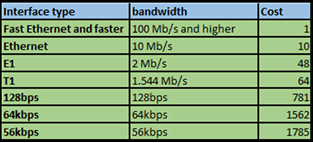# OSPF Cost Calculation Online 2022

## OSPF Cost –

OSPF uses a metric called “Cost” to calculate the metric of the path. The cost is a cumulative value which is an incremental metric.

The cost is as a default based on the bandwidth of the interface. The Higher the interface bandwidth the lower the cost that is associated with that interface.

Related – OSPF LSA Types

To view the cost that is assigned to any given interface which is participating in OSPF, the following command is used –

### Router# show ip ospf interface

The output of this command will show the current cost given to this interface. The costs of the interface is calculated by taking the bandwidth of the interface and dividing this number by a value known as the “auto-cost reference-bandwidth“. This auto-cost reference-bandwidth is an integer used to calculate a standard metric across OSPF and is set to 100,000,000. The cost is calculated as follows:

100,000,000/BW

If the interface bandwidth is 10Mbps, then the resulting cost would be 10:

100,000,000/10,000,000 = 10

If the interface bandwidth is 100Mbps, then the resulting cost would be 1:

100,000,000/100,000,000 = 1

Below table may be referred while calculating the OSPF cost for the interface –The next example reveals some deficiencies with the auto-cost reference-bandwidth set at 100,000,000. If we had a 1Gbp interface it’s cost will be calculated by OSPF to be the exact same number as that for the 100Mbps interface.

100,000,000/1000,000,000 = 1

The same would apply for higher speed interfaces such as 10Gbps interfaces which would also be interpreted by OSPF as having a cost of 1, since OSPF cannot define interface costs as an integer part of a decimal number i.e. 0.5 or 0.125.

So that OSPF may be able to calculate the cost of an interface based on the bandwidth of the interface with great accuracy we will need to change the value of the auto-cost reference-bandwidth value from 100,000,000 to a greater value.

To change the reference bandwidth , we can use the following commands:

router(config)#router ospf 1

R1(config-router)#auto-cost reference-bandwidth 10000

% OSPF: Reference bandwidth is changed.

Note – Please ensure reference bandwidth is consistent across all routers.

The auto-cost reference-bandwidth now has a value of 10000,000,000 (The value is set in Mbps), therefore now the OSPF process will view the 100Mbps interface as having a cost of 100, 1Gbps having a Cost of 10 and 10Gbps a cost of 1, another point worth mentioning is that the router will prompt you to make sure that the auto-cost reference-bandwidth value is set consistently across all your routers.

Note: The cost given to a path is added to the metric only when the route is received inbound on an interface, therefore should you wish to alter the cost of the path you must do this on the inbound interface.

OSPF Timers

Tags: